Learning Library
Who we are
Guidance

# Recipes with Fractions

Recipes with fractions are great for kids learning this important math skill. Cooking helps teach kids practical applications for math, and will help them attain fluency with fractions. Not only that, but cooking is fun! You and your child can mix things up in the kitchen with this fun fraction series. Practice pairing down recipes, using real recipes from our Activities section of the site.

Click on a worksheet in the set below to see more info or download the PDF.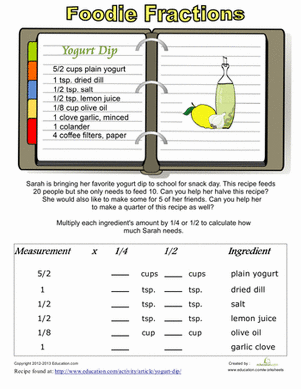### Recipe Conversion

Introduce your little math champ to fraction multiplication in the kitchen! He'll get a taste of measurements using a recipe to calculate the fractions.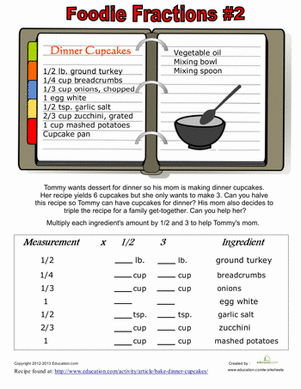### Recipe Fractions #2

Use food and math together to learn fractions! Help your child multiply fractions to convert these recipes to smaller or larger portions.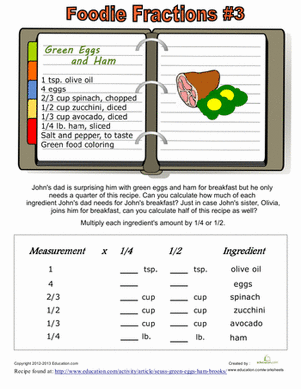### Recipe Fractions #3

Combine math with the fun of cooking! Practice fraction multiplication using the measurements of ingredients from a recipe.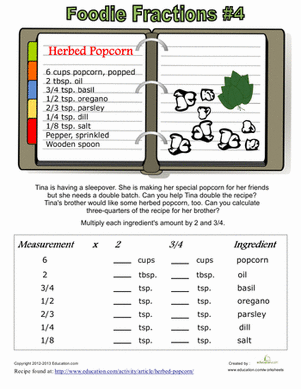### Recipe Fractions #4

Practice multiplying fractions while learning to cook in the kitchen! Your little mathematical chef will adjust the measurements of a real recipe.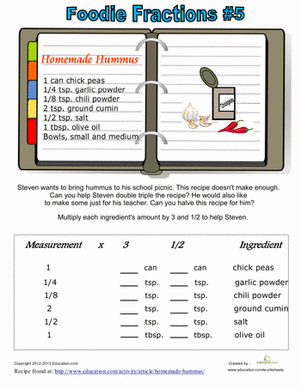### Recipe Fractions #5

Use food to make math fun! Practice multiplying fractions using a recipe. Your little math whiz will adjust the recipe to make more and to make less.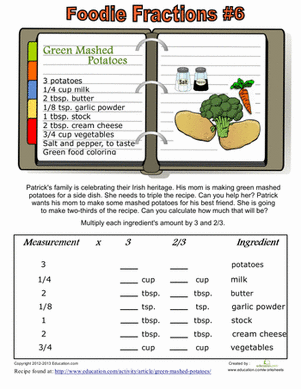### Recipe Fractions #6

Fraction practice can be fun when you add food into the mix! Your child will multiply fractions to change the amounts of ingredients in a recipe.

The set is continued below.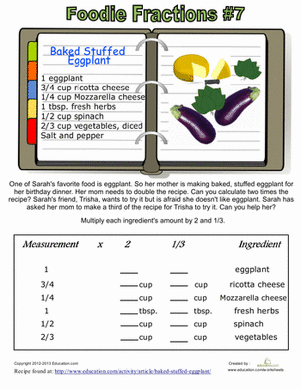### Recipe Fractions #7

Practice multiplying fractions using a real recipe. Your child will have a chance to learn about food as she learns about math.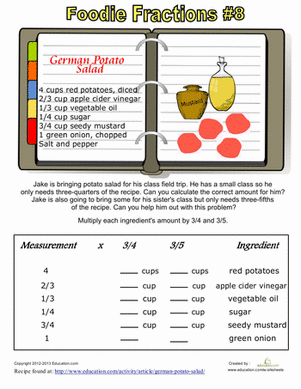### Recipe Fractions #8

Have some fun with fractions in the kitchen! Your little mathematician can be a little chef too, as he practices multiplying ingredient amounts with fractions.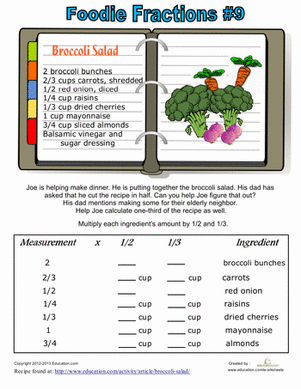### Recipe Fractions #9

Mix math up by combining cooking and fractions! Your little star will practice multiplying fractions that are part of a recipe.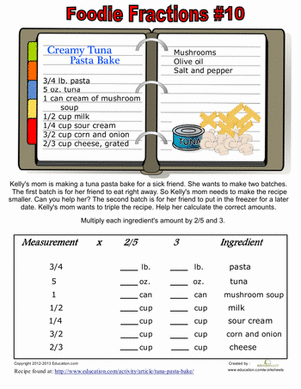### Recipe Fractions #10

Stir up a fun math activity with foodie fraction multiplication! Use a real recipe to practice multiplying fractions.